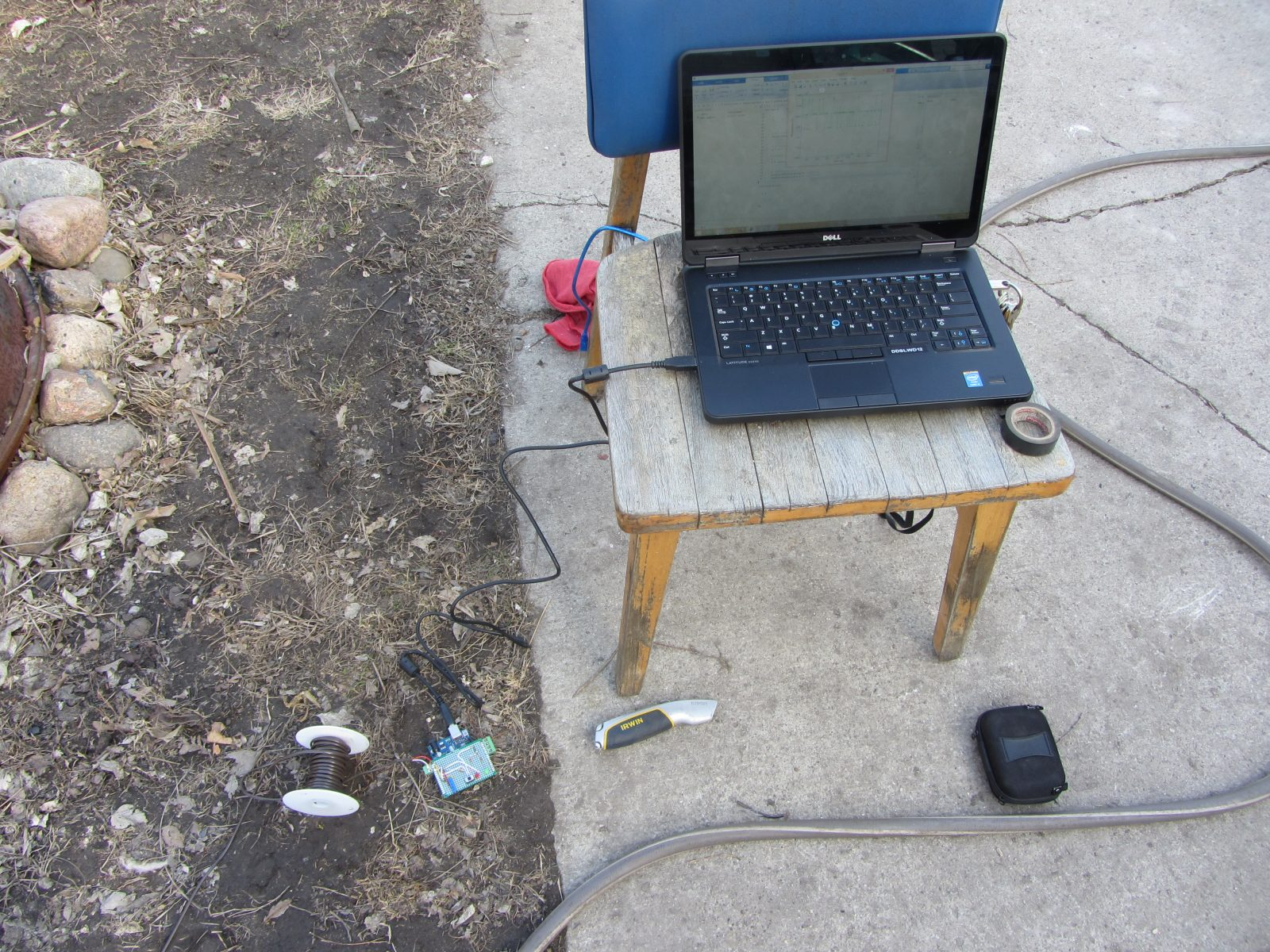# Live Action MATLAB – Arduino data logger.

### Background

I was in need of an affordable data logger for a project. I have access to MATLAB on all of my machines so it was an easy choice to build an Arduino based data logger that would easily interface with MATLAB R2014a. MATLAB is limited in both its sampling frequency (25 Hz) and its time lag (1 sec) but for my purposes these restraints where not a hindrance.

In the fall of 2014 MATLAB updated their Arduino support package. The “Arduino Support from MATLAB” can be found here Unlike prior MATLAB Arduino support packages the user no longer has to upload a script to the Arduino board, this is done automatically by MATLAB when the function “arduino” is run. A full list of the MATLAB – Arduino functions can be found here here### Arduio Shield Layout

The layout of the Arduino was done in Fritzing. This shield allows the data logger setup to log resistance though the means of an adjustable voltage divider.

• 5V power is fed to the sensor to be measured via the screw terminal that is shown on the left side of the schematic. The 5V power is than fed to Analog Port 1 and is measured during the script to obtain the actual voltage going into the voltage divider.
• The 100k trim pot in the lower left acts as the bottom leg of the voltage divider. This is adjustable to allow the data logger to be used with a variety of sensors.
• The switch is used as an interface with MATLAB, when the switch is closed MATLAB is collecting data, when the switch is opened MATLAB exits the data collection for loop in the code and saves the data for post processing.

For More Detail:  Live Action MATLAB – Arduino data logger.